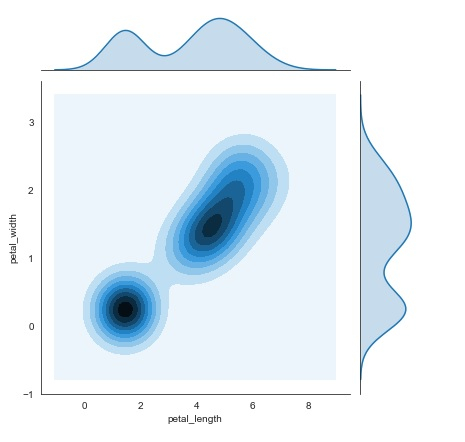# How Seaborn library used to display a kernel density estimation plot (joinplot) in Python?

Seaborn is a library that helps in visualizing data. It comes with customized themes and a high level interface.

Kernel Density Estimation, also known as KDE is a method in which the probability density function of a continuous random variable can be estimated. This method is used for the analysis of the non-parametric values. While using ‘jointplot’, if the argument ‘kind’ is set to ‘kde’, it plots the kernel density estimation plot.

Let us understand how the ‘jointplot’ function works to plot a kernel density estimation in python.

## Example

import pandas as pd
import seaborn as sb
from matplotlib import pyplot as plt
sb.jointplot(x = 'petal_length',y = 'petal_width',data = my_df,kind = 'kde')
plt.show()

## Output## Explanation

• The required packages are imported.

• The input data is ‘iris_data’ which is loaded from the scikit learn library.

• This data is stored in a dataframe.